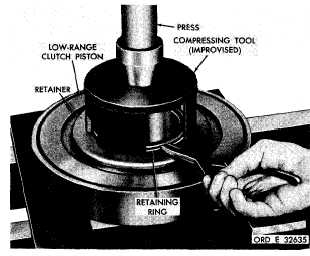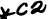Custom Search*C2 *C2 PAR  181-183 L O W ,    R E V E R S E    P I S T O N S    A N D    H O U S I N G    R E B U I LD C H A P   5,   SEC  X X I V i.   Install    spindle    replacer    8351266    on    the planetary   carrier   and   position   the   spindle   for installation    (fig.    217).   Be   sure   that   the   slot   on   the spindle    is    indexed    properly    with    its lock   pin   bore   in   the   carrier   (fig.   217). .   U s i n g     a     p r e s s     a n d     s p i n d l e     r e p l a c er 8351266,   install   the   planetary   carrier   spindle ( f i g .    2 1 7 ) .    T h e    s p i n d l e    r e p l a c e r    w i l l    b o t t om against   the   carrier   when   the   spindle   is   proper- ly   positioned   in   the   carrier. .   Install   the   lock   pin,   using   a   hammer and    punch    (fig.    213).    Drive    the    lock    pin    flush to    0.030-inch    below    the    carrier    surface. .   Install    the    remaining    five    spindles   7 (fig.   377,   fold-out   6)   and   pinions   14,   with      rol- lers   10   and   12,   thrust   washers   8   and   15,   lock pins   16   and   spacers   9,   11   and   13   in   the   same manner   as   described   in   b   through    ,   above. .   Install   bearing   20   in   reverse-range carrier   support   18.   Install   retaining   ring   21. .   I n s t a l l     r e v e r s e - r a n g e     p l a n e t a r y     c a r- r i e r     a s s e m b l y     6     i n     t h e     c a r r i e r     s u p p o r t     1 8. Press   on   the   carrier   assembly   until   it   bottoms in   the   support. S e c t i o n    X X I V .    L O W -    A N D    R E V E R S E - R A N G E    P I S T O N S    A N D    H O U S I N G    —    R E B U I LD 1 8 2 .     D E S C R I P T I O N The   low-   and   reverse-range   clutch   pistons a r e    c o n t a i n e d    i n    a    c o m m o n ,    m a c h i n e d    s t e el h o u s i n g .    T h e    p i s t o n s ,    p i s t o n    r e t u r n    s p r i n g s, retainers,   ring   seals,   seal   ring   expanders   and r e t a i n e r    r i n g s    c o n t a i n e d    i n    t h i s    h o u s i n g    f or low-   and   reverse-range   are   identical   and   inter- c h a n g e a b l e . 1 8 3 .    D I S A S S E M B L Y    ( f i g .    3 7 6 ,    f o l d - o u t    5) .   P o s i t i o n     t h e     l o w -     a n d     r e v e r s e - r a n ge c l u t c h    p i s t o n    h o u s i n g    a s s e m b l y    i n    a    p r e s s (fig.    218). .   U s i n g    a    c o m p r e s s i n g    t o o l    ( f i g .    3 8 ) , remove    the    retaining    ring    retaining    the    piston r e t u r n     s p r i n g     r e t a i n e r     ( f i g .     2 1 8 ) .     R e m o ve the    retainer. .   Install    eighteen    piston    return    springs 49   (fig.   376,   fold-out   5). .   R e m o v e     t h e     l o w - r a n g e     c l u t c h     p i s t on (  f  i  g  .   2  1  8  ) . .   Remove   ring   seal   52   and   expander   51 ( f i g .    3 7 6 ,    f o l d - o u t    5 )    f r o m    l o w - r a n g e    c l u t ch piston   50. .   Remove   ring   seal   53   and   expander   54 from   piston   housing   55 . .   R e m o v e    r e v e r s e - r a n g e    c l u t c h    p i s t on 60,   piston   return   springs   61,   retainer   62,   re- taining   ring   63,   ring   seals   57   and   58,   and   ex- panders   56   and   59   in   the   same   manner   as   de- scribed   for   low-range   in   b   through   ,   a b o v e. Figure   218.   Removing   (or   installing)   retaining   ring for low-range piston return spring retainer 1 4 9Integrated Publishing, Inc. - A (SDVOSB) Service Disabled Veteran Owned Small Business